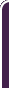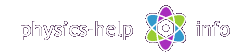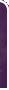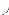homephysics online guidephysics help my info terms of use contactsWhat is Physics? Mechanics Electricity and Magnetism Electric Field Gauss' Law about below subjects Electric Potential Capacity Direct Current Magnetic Field Magnetic Field Laws Magnetic Interactions Electromagnetic Induction Maxwell's Equations Oscillations and Waves Simple Harmonic Motion Damped Harmonic Motion Driven Harmonic Motion Electric Oscillation Alternating Current Wave Motion Elastic Waves Electromagnetic Waves Optics Light Waves Geometrical Optics Interference Polarization Diffraction Fraunhofer Diffraction Dispersion, Absorption, Diffusion Doppler Effect Thermodynamics Ideal Gas Molecular Statistics Transport Phenomena First Law of Thermodynamics Second and Third Laws of Thermodynamics Imperfect Gas Liquids Solids Quantum Physics Thermal Radiation Quantum Properties of Light Wave Properties of Particles Planetary Model of Atom X-Rays Particle in Potential Well Pauli Exclusion Principle Nuclear Physics Solid State Physics Appendices# Work and Energy

SI units & Physics constants

Work and energy investigates different forms of energy, their transmutation, and interrelationship between energy and workHere (all units see here):

m is mass of objectis force vectoris displacement vectoris angle between vectorsandis velocity

## General formulas

Work done by constant force during small displacement is defined by scalar productWork done by force during motion between initialand finalpositions is defined by definite integral over trajectoryInstantaneous power is defined as derivative with respect to timeAverage power required to perform work W during time tRelation between power, force and velocityKinetic energy of objectConservative force is the force when the work it does on object is independent of the path between the object's initial and final positions. Otherwise the force is called nonconservative

Potential energy, Ep, for conservative force is defined by relationwhere:

W is work done by the force

Epi  and Epf are potential energies at initial and final positions respectively

Gravitational potential energy of object at height hwhere g is free-fall acceleration

Total mechanical energy## Work-Energy Theorem

General Work-Energy theoremwhere:

W is net work done by external nonconservative forces acting on the system

Eki, E pi are initial kinetic and potential energies respectively

Ekf, E pf are final kinetic and potential energies respectively

Principal of conservation of energy for conservative system (without nonconservative forces)## Work and energy of spring

Work done by springwhere:

k is spring constant

xo is length of unstretched spring

xi and xf are initial and final lengths of the spring respectively

Potential energy of spring stretched to length xEnergy conservation principal for spring-mass systemwhere vi and vf are initial and final speeds of the mass respectively

## Work and energy of rotational motion

Work done by torquewhere:is component of net torque parallel to axis of rotationandare initial and final angular positions of object

Kinetic energy of rotating objectwhere:

I is moment of inertia of object about its axis of rotationis angular speed

Total kinetic energy of moving objectwhere:

vc is linear speed of mass center of the objectis angular speed of the object

m is mass of the object

Ic is moment of inertia of the object about the axis of rotation passing through its center of masshome  |  physics online guide  |  physics help  |  my info  |  terms of use  |  contacts Dr. Nikitin © 2010